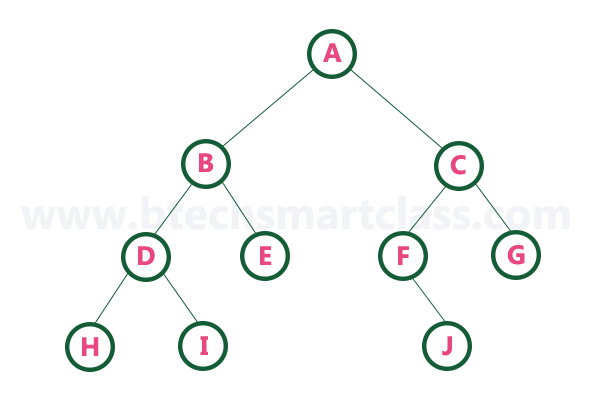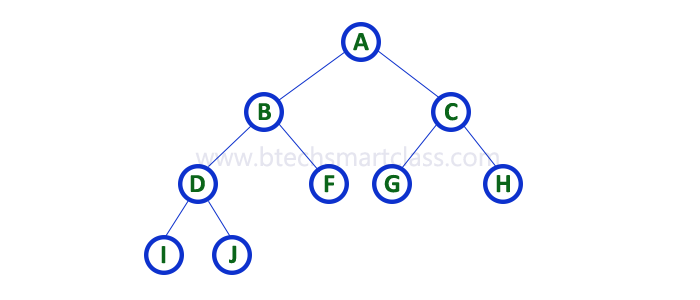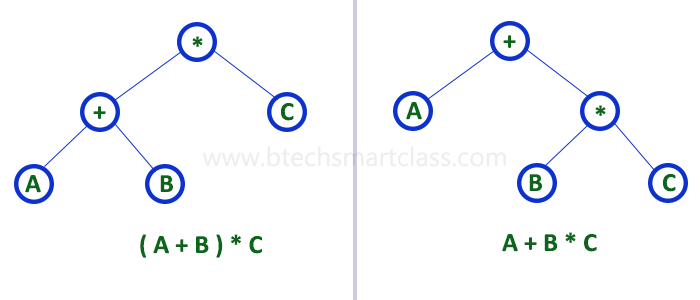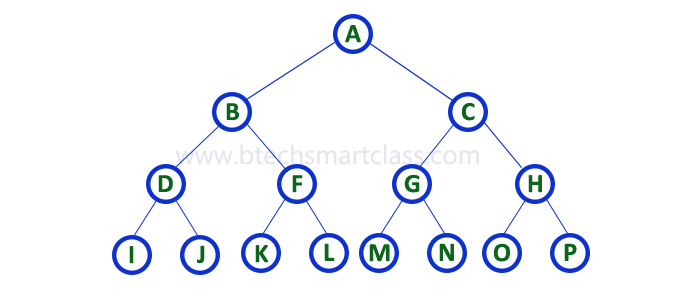The perfect place for easy learning...

×

# Binary Tree Datastructure

In a normal tree, every node can have any number of children. A binary tree is a special type of tree data structure in which every node can have a maximum of 2 children. One is known as a left child and the other is known as right child.

A tree in which every node can have a maximum of two children is called Binary Tree.

In a binary tree, every node can have either 0 children or 1 child or 2 children but not more than 2 children.

##### ExampleThere are different types of binary trees and they are...

# 1. Strictly Binary Tree

In a binary tree, every node can have a maximum of two children. But in strictly binary tree, every node should have exactly two children or none. That means every internal node must have exactly two children. A strictly Binary Tree can be defined as follows...

A binary tree in which every node has either two or zero number of children is called Strictly Binary Tree

Strictly binary tree is also called as Full Binary Tree or Proper Binary Tree or 2-TreeStrictly binary tree data structure is used to represent mathematical expressions.

##### Example# 2. Complete Binary Tree

In a binary tree, every node can have a maximum of two children. But in strictly binary tree, every node should have exactly two children or none and in complete binary tree all the nodes must have exactly two children and at every level of complete binary tree there must be 2level number of nodes. For example at level 2 there must be 22 = 4 nodes and at level 3 there must be 23 = 8 nodes.

A binary tree in which every internal node has exactly two children and all leaf nodes are at same level is called Complete Binary Tree.

Complete binary tree is also called as Perfect Binary Tree# 3. Extended Binary Tree

A binary tree can be converted into Full Binary tree by adding dummy nodes to existing nodes wherever required.

The full binary tree obtained by adding dummy nodes to a binary tree is called as Extended Binary Tree.In above figure, a normal binary tree is converted into full binary tree by adding dummy nodes (In pink colour).